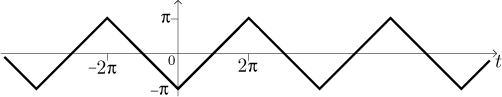Problem: For the following function find its Fourier series, its sine Fourier series and its cosine Fourier series; for each series, determine its sum.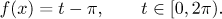Solution: This seems to be a standard problem, so we use the usual procedure.

Fourier series:
When we extend the given function periodically, its period will be T = 2π. The corresponding frequency is therefore ω = 1. Now we substitute into appropriate formulas, two of the three integrals have to be evaluated using integration by parts.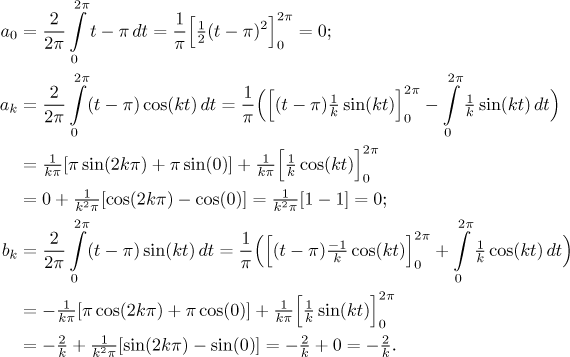Thus we get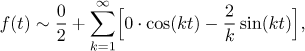that is,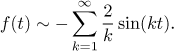To find the sum of this series we use Jordan's conditions. First we draw the periodic extension of the given function f and then at all points of discontinuity we place dots at levels that average left and right limits there.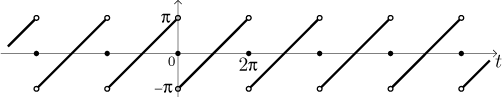Sine Fourier series:
Actually, the Fourier series consists only of sines, so it is already a sine series. Indeed, the periodic extension that we drew above was an odd function, so this is automatic.

What would happen if we applied the standard procedure for obtaining sine Fourier series? We would eventually get the same answer.

We get the sine Fourier series by considering an extension of the given function f to [−2π,0) in such a way that the resulting function is odd. The original function has the length of domain L = 2π, so the doubled function will form periodic extension with T = 2L = 4π and half the original frequency, ω = 1/2. We know that if we apply the standard procedure to this new setup, then the formulas simplify so that eventually we can use the usual formulas with L in place of T, applied to the original interval, but with the new ω. Obviously, for a sine series the coefficients a0 and ak are automatically zero and we only need to evaluate bk.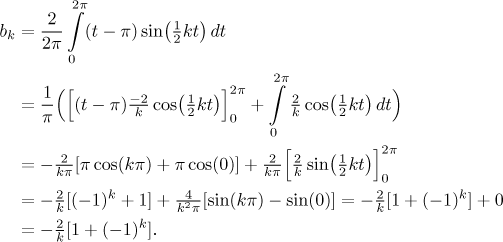Thus we get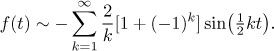Note that the expression 1 + (−1)k is equal to 0 for k odd, so odd multiples actually disappear from this series. For even k, this expression becomes equal to 2. Thus we can rewrite this series just for even multiples by replacing k with 2k.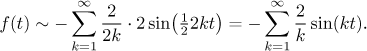We obtained the same series as above. To determine its sum we would start by drawing an odd periodic extension of f, but note that in the picture above, the extension of f is already odd, so that picture still applies.

Cosine Fourier series:
Now we apply the standard procedure to find the cosine Fourier series. We get it by considering an extension of the given function f to
[−2π,0) in such a way that the resulting function is even. The original function has the length of domain L = 2π, so the doubled function will form periodic extension with T = 2L = 4π and half the original frequency, ω = 1/2. Again, we know that if we apply the standard procedure to this new setup, then the formulas simplify, this time the coefficients bk are automatically zero and we need to evaluate a0 and ak.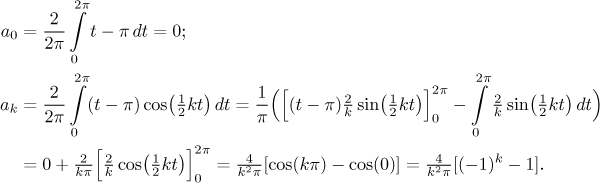Thus we get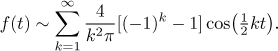Note that the expression (−1)k − 1 is equal to 0 for k even, so even multiples actually disappear from this series. For odd k, this expression becomes equal to −2. Thus we can rewrite this series just for odd multiples by replacing k with 2k + 1. In order to get all odd positive integers (including 1), we now have to start indexing from 0.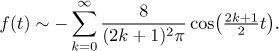To determine the sum of this series we start by drawing an even periodic extension of f, then we should handle points of discontinuity, but since there are none, we know that the cosine Fourier series converges to this extension and this convergence is uniform on the real line.